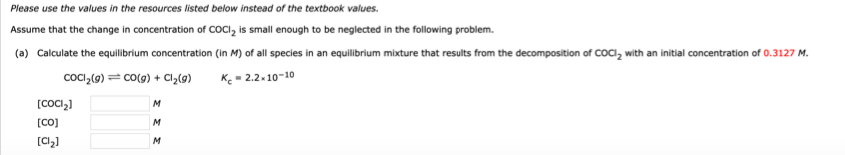# Please use the values in the resources listed below instead of the textbook values. Assume that the change in concentration of COCl2 is small enough to be neglected in the following problem. (a) Calculate the equilibrium concentration (in M) of all species in an equilibrium mixture that results from the decomposition of COCl2 with an initial concentration of 0.3127 M. COCl2(g) CO(g) + C12(g) Kc = 2.2 x 10-10 [C0C12] [CO] [Cl2] (b) Show that the change is small enough to be neglected. Since the percent error between the equilibrium and the initial concentration is be neglected.